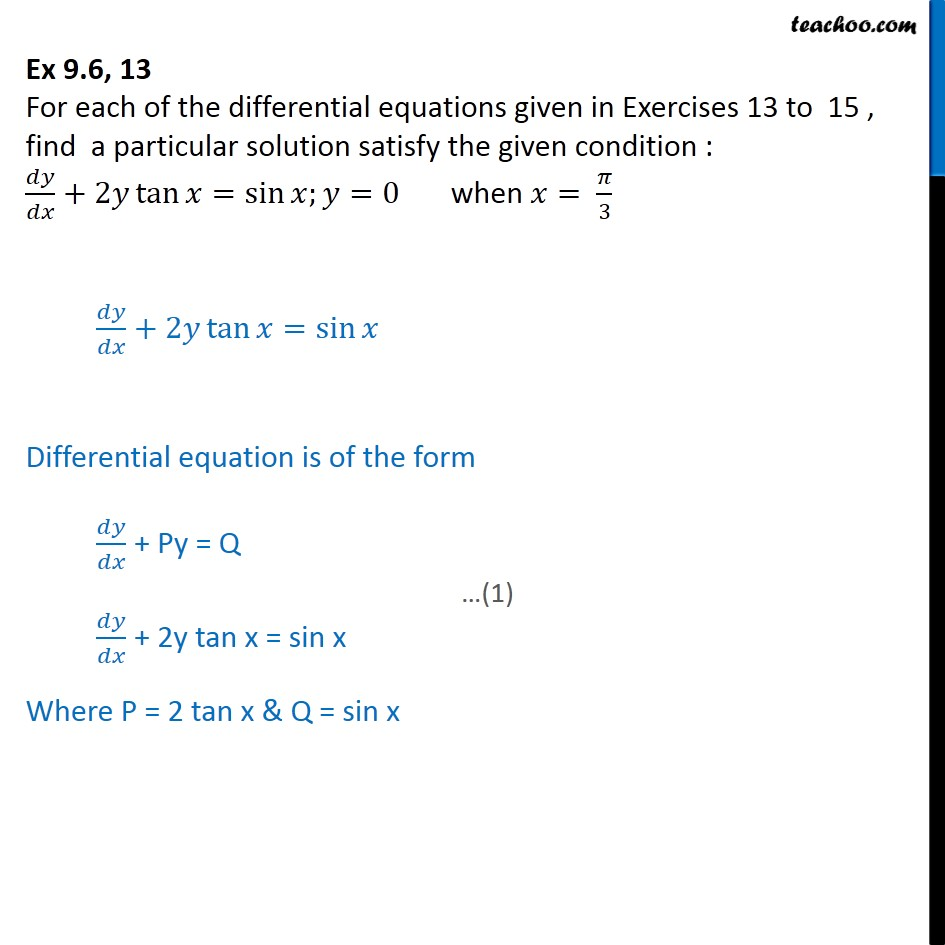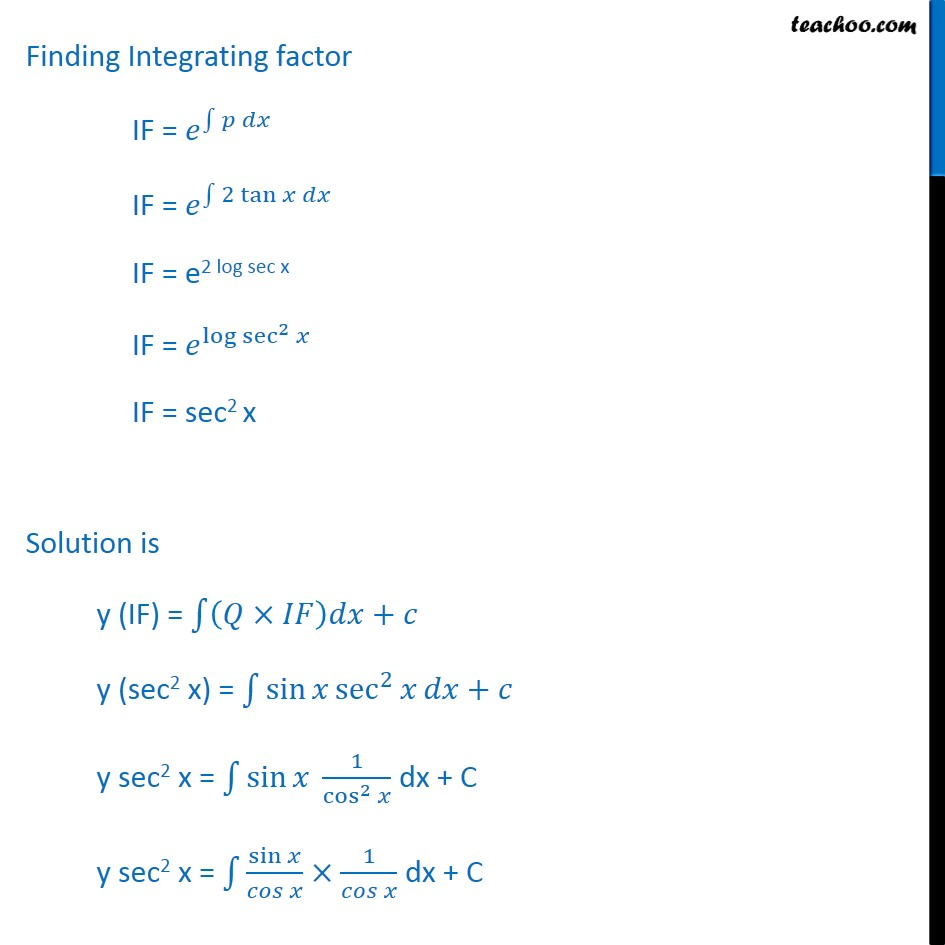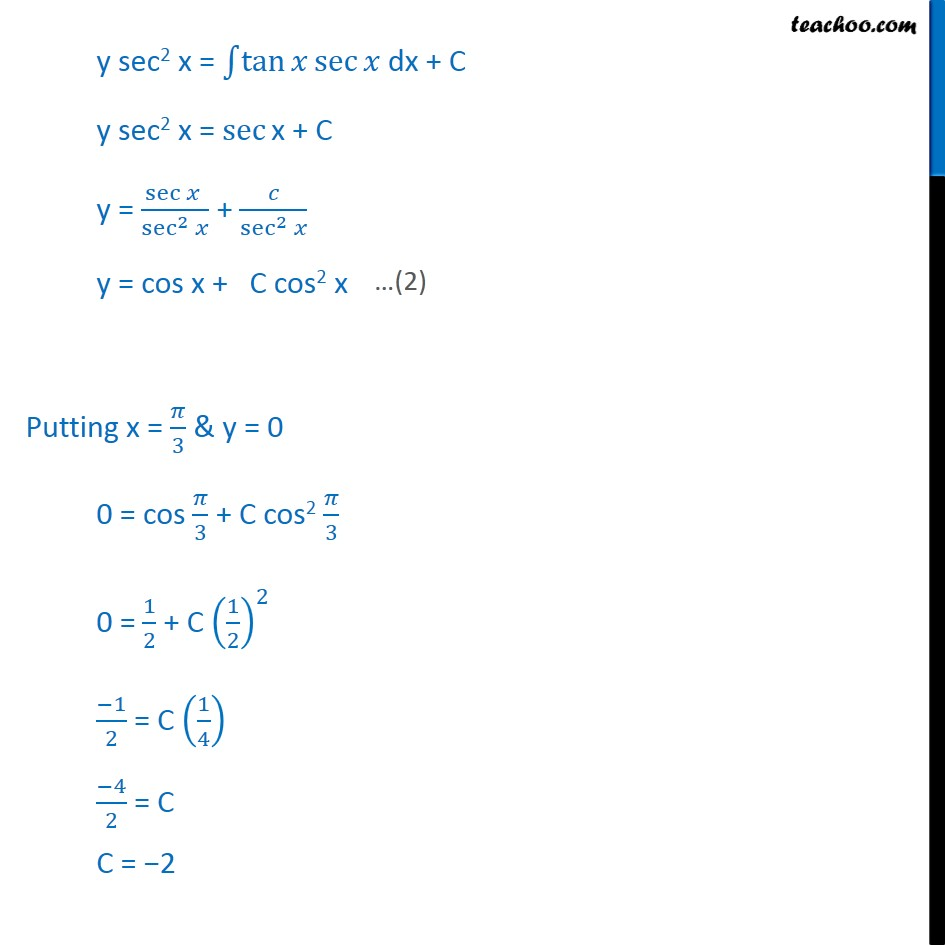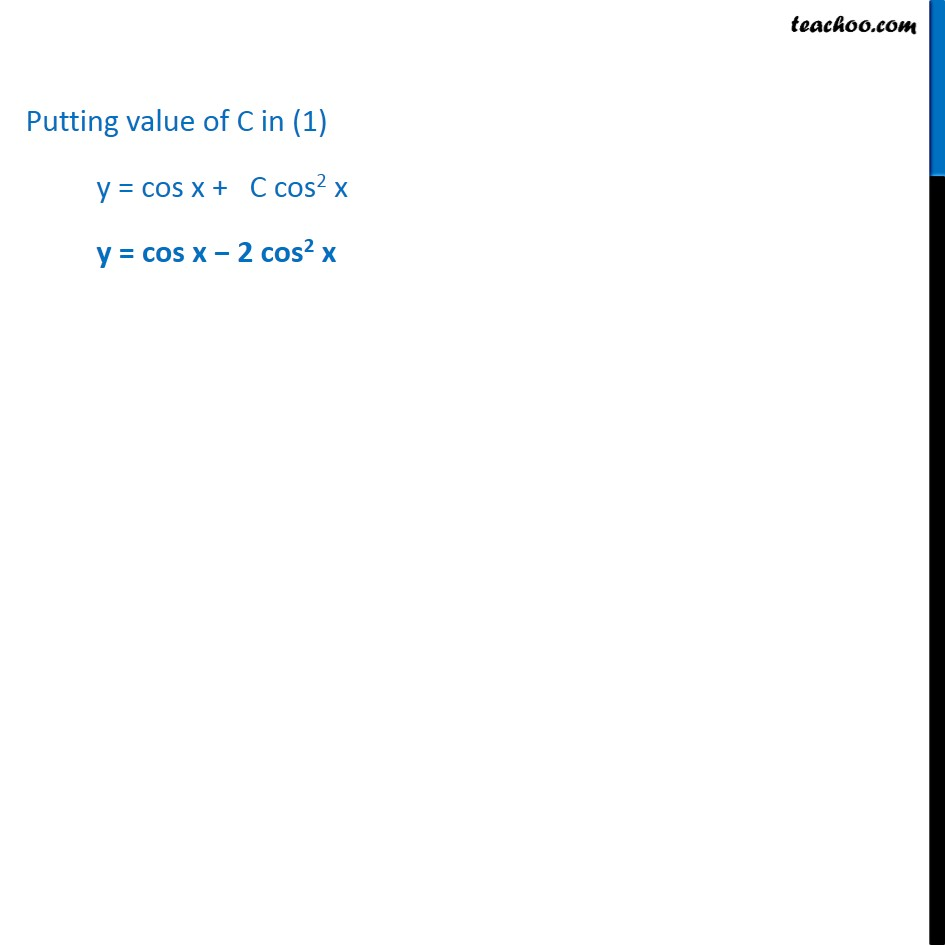Ex 9.5

Chapter 9 Class 12 Differential Equations
Serial order wiseLearn in your speed, with individual attention - Teachoo Maths 1-on-1 Class

### Transcript

Ex 9.5, 13 For each of the differential equations given in Exercises 13 to 15 , find a particular solution satisfy the given condition : 𝑑𝑦/𝑑𝑥+2𝑦 tan⁡〖𝑥=sin⁡〖𝑥;𝑦=0〗 〗 when 𝑥= 𝜋/3 𝑑𝑦/𝑑𝑥+2𝑦 tan⁡〖𝑥=sin⁡𝑥 〗 Differential equation is of the form 𝑑𝑦/𝑑𝑥 + Py = Q 𝑑𝑦/𝑑𝑥 + 2y tan x = sin x Where P = 2 tan x & Q = sin x Finding Integrating factor IF = 𝑒^∫1▒〖𝑝 𝑑𝑥〗 IF = 𝑒^∫1▒〖2 tan⁡𝑥 𝑑𝑥〗 IF = e2 log sec x IF = 𝑒^log⁡sec^2⁡𝑥 IF = sec2 x Solution is y (IF) = ∫1▒〖(𝑄×𝐼𝐹)𝑑𝑥+𝑐〗 y (sec2 x) = ∫1▒〖sin⁡𝑥 sec^2⁡𝑥 𝑑𝑥+𝑐〗 y sec2 x = ∫1▒〖sin⁡𝑥 1/cos^2⁡𝑥 〗 dx + C y sec2 x = ∫1▒〖sin⁡𝑥/𝑐𝑜𝑠⁡𝑥 ×1/𝑐𝑜𝑠⁡𝑥 〗 dx + C y sec2 x = ∫1▒tan⁡〖𝑥 sec⁡〖𝑥 〗 〗 dx + C y sec2 x = sec⁡"x + C " y = sec⁡〖𝑥 〗/sec^2⁡𝑥 + 𝑐/sec^2⁡𝑥 y = cos x + C cos2 x Putting x = 𝜋/3 & y = 0 0 = cos 𝜋/3 + C cos2 𝜋/3 0 = 1/2 + C (1/2)^2 (−1)/2 = C (1/4) (−4)/2 = C C = −2 Putting value of C in (1) y = cos x + C cos2 x y = cos x − 2 cos2 x